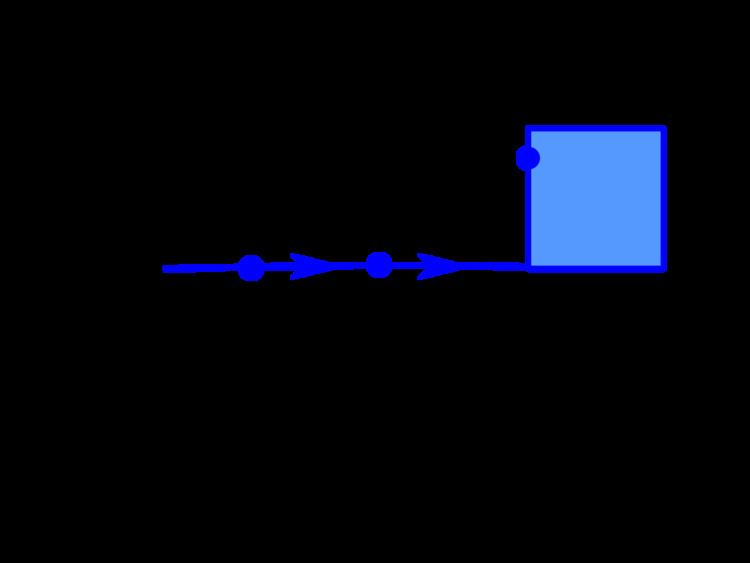# Hemicontinuity

Updated onIn mathematics, the notion of the continuity of functions is not immediately extensible to multi-valued mappings or correspondences between two sets A and B. The dual concepts of upper hemicontinuity and lower hemicontinuity facilitate such an extension. A correspondence that has both properties is said to be continuous in an analogy to the property of the same name for functions.

## Contents

Roughly speaking, a function is upper hemicontinuous when (1) a convergent sequence of points in the domain maps to a sequence of sets in the range which (2) contain another convergent sequence, then the image of limiting point in the domain must contain the limit of the sequence in the range. Lower hemicontinuity essentially reverses this, saying if a sequence in the domain converges, given a point in the range of the limit, then you can find a sub-sequence whose image contains a convergent sequence to the given point.

## Upper hemicontinuity

A correspondence Γ : AB is said to be upper hemicontinuous at the point a if for any open neighbourhood V of Γ(a) there exists a neighbourhood U of a such that for all x in U, Γ(x) is a subset of V.

## Sequential characterization

For a correspondence Γ : AB with closed values, Γ : AB is upper hemicontinuous at a A if a n A , b B and b n Γ ( a n )

lim n a n = a , lim n b n = b b Γ ( a )

If Γ is compact-valued (i.e. Γ(x) is compact for all x) the converse is also true.

## Closed Graph Theorem

The graph of a correspondence Γ : AB is the set defined by G r ( Γ ) = { ( a , b ) A × B : b Γ ( a ) } .

If Γ : AB is an upper hemicontinuous correspondence with closed domain (that is, the set of points aA where Γ(a) is not the empty set) and closed values (i.e. Γ(a) is closed for all a in A), then Gr(Γ) is closed. If B is compact, then the converse is also true.

## Lower hemicontinuity

A correspondence Γ : AB is said to be lower hemicontinuous at the point a if for any open set V intersecting Γ(a) there exists a neighbourhood U of a such that Γ(x) intersects V for all x in U. (Here V intersects S means nonempty intersection V S ).

## Sequential characterization

Γ : AB is lower hemicontinuous at a if and only if

a m A , a m a , b Γ ( a ) , a m k subsequence of a m , b k Γ ( a m k ) , b k b

## Open Graph Theorem

A correspondence Γ : AB have open lower sections if the set Γ 1 ( b ) = { a A : b F ( a ) } is open in A for every bB. If Γ values are all open sets in B, then Γ is said to have open upper sections.

If Γ has an open graph Gr(Γ), then Γ has open upper and lower sections and if Γ has open lower sections then it is lower hemicontinuous.

The open graph theorem says that if Γ : A → P(Rn) is a convex-valued correspondence with open upper sections, then Γ has an open graph in A × Rn if and only if Γ is lower hemicontinuous.

## Properties

Set-theoretic, algebraic and topological operations on multivalued maps (like union, composition, sum, convex hull, closure) usually preserve the type of continuity. But this should be taken with appropriate care since, for example there exists a pair of lower hemicontinuous correspondences whose intersection is not lower hemicontinuous. This can be fixed upon strengthening continuity properties: if one of those lower hemicontinuous multifunctions has open graph then their intersection is again lower hemicontinuous.

Very important part of set-valued analysis (in view of applications) constitutes the investigation of single-valued selections and approximations to multivalued maps. Typically lower hemicontinuous correspondences admit single-valued selections (Michael selection theorem, Bressan-Colombo directionally continuous selection theorem, Fryszkowski decomposable map selection), likewise upper hemicontinuous maps admit approximations (e.g. Ancel-Granas-Górniewicz-Kryszewski theorem).

## Implications for continuity

If a correspondence is both upper hemicontinuous and lower hemicontinuous, it is said to be continuous. A continuous function is in all cases both upper and lower hemicontinuous.

## Other concepts of continuity

The upper and lower hemicontinuity might be viewed as usual continuity:

Γ : AB is lower [resp. upper] hemicontinuous if and only if the mapping Γ : AP(B) is continuous where the hyperspace P(B) has been endowed with the lower [resp. upper] Vietoris topology.

(For the notion of hyperspace compare also power set and function space).

Using lower and upper Hausdorff uniformity we can also define the so-called upper and lower semicontinuous maps in the sense of Hausdorff (also known as metrically lower / upper semicontinuous maps).

Similar Topics